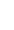# PHYS 100 Chapter Notes - Chapter 8: Omv, One Direction, Inelastic Collision

66 views7 pages
School
Department
Course
ProfessorChapter 8
Momentum:
- Momentum is the tendency of an object to keep moving once it is in motion
- To change the momentum of an object, it will require a force for a certain duration
Linear Momentum
- Linear Momentum and Force
o Defined as the product of a systems mass multiplied by its velocity
P = mv
Momentum is proportional to the objects mass and velocity
Greater an objects mass or greater is velocity, the greaeter the momentum
Momentum (p) is a vector having the same direction as the
velocity (V)
Example: calculate the momentum of a 110kg football plater running at
8m/s
Compare momentum with a football thrown. 0.410kg at 25m/s
P = mv
110kg(8m/s) = 880kg m/s
P = mv
0.410kg(25m/s) = 10.3kg m/s
PPlayer/PFootball = (880kg m/s) / (10.3kg m/s)
85.9
- Momentum and Newtons Second Law
o The net external force equals the change in momentum of a system divided by the
time over which it changes
Fnet = ΔP/Δt
o Example: what is the average force exerted by a 0.057kg ball at the speed of 58
m/s, initial horizontal component is negligible and ball remained in contact with
racquiet for 5.0ms (Miliseconds)
FNet = ΔP/Δt
ΔP = m(Vf Vi)
0.057kg(58m/s 0m/s)
3.3kg m/s
FNet = ΔP/ΔT
ΔP = 3.3kg m/s
ΔT = 5x10-3
= 660N
Angular Momentum:
- The product of the moment of intertia * Angular velocity
o Angular Velocity to express how fast it is working
o L = I w
o I = ½ mr2
o W = v/r
find more resources at oneclass.com
find more resources at oneclass.com
Unlock document

This preview shows pages 1-2 of the document.
Unlock all 7 pages and 3 million more documents.Impulse
- A small force could cause the same change in momentum
o Example: if a ball were to thrown up, the gravitational force would reverse
momentum of the ball
Δp = FnetΔt
FnetΔT is given the name impulse
o The same as the change in momentum
- Change in momentum equals the average net external force multiplied by the time this
force acts upon
o Two bilalrds strike a wall with the same speed, reflected without any change of
speed. First ball strikes perpendicular to the wall, second at 30 degrees from the
perpendicular and bounces off at an angle of 30 degrees from perpendicular to the
wall
Determine the direction of the force
Calculate the ratio of the magnitudes of impulses on the two balls by the
wall
Pxi = mu
o muCos30
o muSin30
Pxf = mu
o muCos30
o muSin30
(2mu)/(2mu cos 30) = 2/3
o 1.155
Conservation of Momentum
- Momentum is an important because it is conserved
o Ptot = Constant
o Ptot = P’tot (Isolated)
Ptot = the total momentum (Sum of the momenta of the individual objects)
P’tot = total momentum some time later (Momentum of the centre of mass)
o Momentum is conserved for an isolated system is to consider Newton’s second
law in terms of momentum
FNet = (ΔPtot)/(Δt)
For an isolated system
o Fnet = 0
o ΔPtot = 0
o Ptot is constant
Elastic Collisions in One Dimesion
- An elastic collision is one that also conserves internal kinetic energy
o Internal kinetic energy is the sum of the kinetic energies of the objects in the
system
find more resources at oneclass.com
find more resources at oneclass.com
Unlock document

This preview shows pages 1-2 of the document.
Unlock all 7 pages and 3 million more documents.